# Fluids in Motion

Idea: Since the motion of fluids can be very complicated (due to turbulence, frictional forces between molecules etc.) we need to make the following simplifying assumptions:

• No internal friction between layers of fluid (zero viscosity).
• The density of the fluid remains the same throughout the fluid (non-compressibility).
• Fluid velocity and pressure at each point in the fluid do not change with time (steady state).
• The fluid particles follow smooth, predictable flow lines (streamline or laminar flow).
Definition: Flow Rate

The flow rate at each point along a (non-uniform) pipe or hose is defined as the volume of fluid passing through the pipe at that point per unit time:

 FlowRate =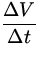= A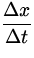= Av (15)

where A is the cross-sectional area of the pipe at that point and v =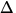x/t is the fluid velocity.

Idea: If the fluid is incompressible, the flow rate must be the same everywhere along the pipe (Conservation of fluid particles: what goes in must come out). This leads directly to the Continuity Equation:

 Av = constant. (16)

Idea: Conservation of energy requires that the work done in moving a volume of fluid through a portion of pipe must go into kinetic energy and/or potential energy of the fluid (assuming no frictional forces). Consider a fluid moving through a pipe as in Fig.9.8.The work done by the pressure P1 on the left in moving the fluid a distancex1 is:

W1 = F1x1 = P1A1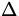x1.

On the right, the work done by the pressure is:

W2 = - F2x2 = - P2A2x2

where the minus sign occurs because the force is in the opposite direction of the motion in this case. By the continuity equation, the amount of fluid entering the pipe on the left must equal the amount leaving on the right. Thus:

A1x1 = A2x2.

This volume of fluid, which we call V , can undergo both a change in kinetic energy and a change in potential energy as it is pushed through the pipe:KE =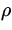Vv22 -Vv12PE =Vgy2 -Vgy1.
Equating the work done ( W = W1 + W2 ) to the change in mechanical energy (KE +PE ) leads directly to Bernouilli's Equation (note that the volume V = A1x1 = A2x2 cancels):

 P1 +v12 +gy1 = P2 +v22 +gy2 (17)

where P1 , v1 and y1 are the pressure, fluid velocity and height of the fluid at one end, etc.

Note: If there is no height difference, Bernouilli's equation relates the pressure at two points along the flow to the fluid velocities:

 P1 - P2 =(v22 - v12). (18)

Thus, pressure in the fluid decreases whenever the fluid velocity increases. This results in important observable effects, such as the lift produced by an airplane wing, the curving of a spinning baseball and the fact that the shower curtain gets sucked in when you first turn on the shower.Next: Problems Up: Solids and Fluids Previous: Buoyant Forces